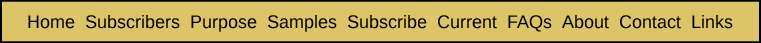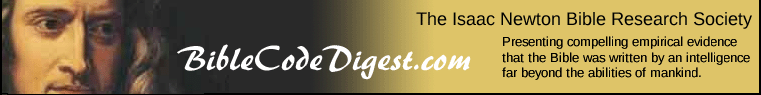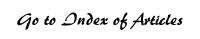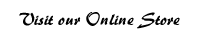A Methodology for Estimating the Combined Probability That a Cluster "Similar" to the One Located Could Appear by Coincidence Appendix Seven from Breakthrough: Encountering the Reality of the Bible Codes It may be helpful to quickly review the overall methodology described in this book for calculating the probability of chance appearance of a given cluster of ELSs. We first determine the expected number of times that each ELS in a cluster could appear by chance in the entire search text (be it the Torah or the entire Tanakh or some section of either). This is determined by applying a formula that takes into account the letter probabilities of the letters that are in the ELS, as well as the size of the skips between successive letters. We then select the ELS that is the most unlikely to appear by chance as the focal ELS of the cluster. The method of calculating the probability that a given cluster of ELSs could appear by chance presented in this book first determines the probability that each ELS could be as close to the focal ELS as it actually is. The product of all these individual probabilities then becomes the probability that the given cluster could appear by chance. This is not really the kind of probability that is key to the question of whether the cluster is real or not, however. What is critical is determining the probability that the given cluster, or one judged to be "similar," could appear by chance. By similar we mean that each of the alternative ELSs in the similar cluster would have a comparable probability of chance appearance. Any alternative ELS could be judged to be similar as long as it was considered to be equally relevant to the topic of the cluster and its probability was similar. Its probability could be similar even though it might have fewer letters, as long as the size of its skips was enough shorter and its location relative to the focal code were such that its probability of chance appearance was close to that of the ELS that was originally located. The probability of chance appearance of each located code is modified to reflect the fact that we could have found some number N of other ELSs that would be similar to the one we did find. Finally, by taking the product of these adjusted probabilities for each ELS, we obtain the probability that a cluster could appear by chance that had ELSs similar to those actually in the cluster. How would the number of ELSs (N) similar to each located ELS be determined? First we select an estimate for the total number of ELSs of one word that we would agree could be relevant to the specific topic described by the ELSs in the cluster. Suppose we agree on 5,000. This would include possible alternative spellings of each located ELS or of alternative words judged to be equally relevant to the topic of the cluster. Then we count up the number of ELSs we have already located in the cluster. Suppose we have 50. Since we have already found 50, that means there could be 100 ( = 5,000 / 50 ) similar ELSs for each one we have already found. Or more precisely, there are 99 similar ELSs for each one we have already found, since the one we already found should be included in the 100. In Appendices Two through Six, we have presented a series of formulae that can be used to determine the probability that any given ELS could cross a specified section of text by chance. In this appendix, we will cover the mathematical basis for transforming that kind of probability into one for the likelihood that the given ELS, or any one of (N – 1) similar ELSs, could cross the specified section of text by chance. We will begin by assuming that the probability of an ELS crossing a given section of text can be closely approximated by the Poisson distribution. Given that,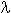is the expected number of crossing ELSs, and the probability of any given number (k) of crossings, given that its expected number of crossings is E, is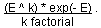Now the probability that none of the ELSs cross the section may be obtained by setting k=0 for the Poisson distribution. This gives us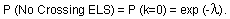Since all possible events may be grouped into one of two categories: (1) none of the ELSs cross the section, or (2) one or more ELSs cross the section, this gives usFrom this we obtain the following: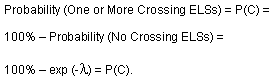This gives us Formula 7A.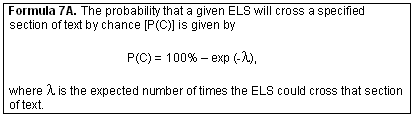Having this formula, we can rearrange it so that we can calculate the expected number of crossings () from the probability that one or more ELSs cross the section of text (P(C)). We proceed as follows: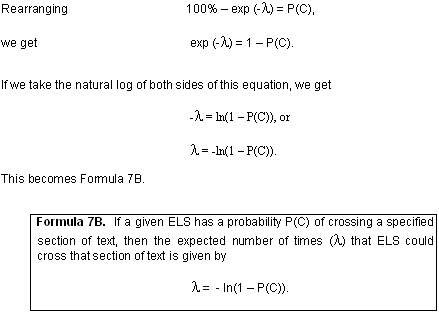Now, the sum of N different Poisson distributions is also a Poisson distribution with a lambda value that is the sum of the lambda values of each of the N distributions. For a given ELS we have located, each similar ELS will have a lambda value approximately equal to that of the original ELS. Therefore, as a basic approximation, we have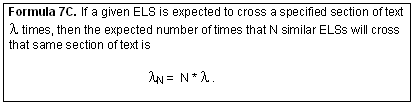By substituting Formula 7C into Formula 7A, we have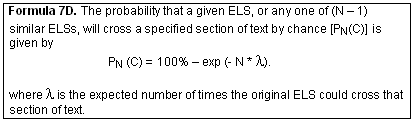While we have assumed that each of these probability distributions may be approximated by the Poisson distribution, we would note that empirical testing we have performed has verified that this assumption is valid, subject to random variation due to sampling error.

 Enjoy finding your own Bible codes. Bible code search software is available in our online store. Subscribe Free! By signing up to be a member of The Isaac Newton Bible Research Society, you will have access to more than fifteen years of research by our team of Bible code researchers. Sign up to be a member today.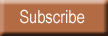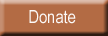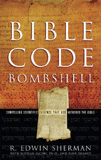Bombshell examines two massive, recently discovered clusters of codes in the Hebrew Old Testament. To read more about Bombshell, click here, or click below to order from Amazon today!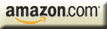| HOME | SUBSCRIBERS ONLY | OUR PURPOSE | FREE SAMPLES | CURRENT | | FAQs | ABOUT BIBLE CODES | SUBSCRIBE | CONTACT | Copyright © 2016 BibleCodeDigest.com